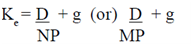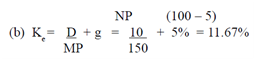## Dividend yield plus growth in dividend method, Financial Management

Assignment Help:

Dividend yield plus growth in dividend method

When the dividends of the firm are predictable to grow at a constant rate and the dividend payout ratio is constant, this technique may be used for calculating the cost of equity shares.Where, Ke = cost of Equity capital, D = Expected dividend per share, g = rate of growth in dividends, MP = Market price of equity shares and NP = Net proceeds per share

Illustration:

A Co. plans to issue 1000 new shares of Rs.100 each at par.  The floatation costs are expected to be 5% of the share price. The co. pays a dividend of Rs.10 per share initially and the growth in dividends is expected to be 5%. (a) Compute the cost of new issue of equity shares. (b) If the current market price of an equity share is Rs.150, calculate the cost of existing equity share capital

Solution: (a)  K = D + g =        10  + 5% = 15.53%#### return on equity, Lee Sun's has sales of \$6,000, total assets of \$5,000, a...

Lee Sun's has sales of \$6,000, total assets of \$5,000, and a profit margin of 10 percent. The firm has a total debt ratio of 40 percent. What is the return on equity?

#### Performance evaluation, Performance evaluation One can determine this b...

Performance evaluation One can determine this by comparing the cash flow from assets and cost of capital. 1. Cash flow from assets Cash flow from assets is calculated

#### Define which is lower cost of debt or cost of equity, Which is lower for a ...

Which is lower for a given company:  the cost of debt or the cost of equity?  Explain: Ignore taxes in your answer . The cost of debt is all the time less as compared to the cost

#### Valuing semi-annual cash flows, In all previous illustrations, we ass...

In all previous illustrations, we assumed that coupon payments are paid on annual basis. However, most of the bonds carry interest payment semi-annually. Semi-ann

#### Solution to shareholders versus managers conflict, Solutions to this Confli...

Solutions to this Conflict In common, to make sure that managers act to the best interest of shareholders, the firm will: (a) Acquire Agency Costs in the form of:

#### Accounting for financial instruments, The IASB is in the procedure of under...

The IASB is in the procedure of undertaking a comprehensive review of accounting for financial instruments, and has issued a latest financial instruments standard referred to as IF

#### Determine rates that company enter into a \$/£ currency swap, Suppose a comp...

Suppose a company is quoting swap rates as follows:  7.75 - 8.10 percent yearly against 6-month dollar LIBOR for dollars and 11.25 - 11.65 percent yearly against six-month dollar L

#### Return on common equity finance basics, At the end of the fiscal year endin...

At the end of the fiscal year ending June 30, 2003, Microsoft reported common equity of \$64.9 billion on its balance sheet, with \$49.0 billion invested in financial assets (in the

#### Determine the factors of financial risk by giving example, Determine the fa...

Determine the factors of financial risk by giving example W. T. L. Company's cost of long-term debt two years ago was 8 percent.  This 8 percent was found to represent a 4- per

#### CASELET, Prepare your recommendation on Agarwal Cast Company

Prepare your recommendation on Agarwal Cast Company